## Loren on the Art of MATLABTurn ideas into MATLAB

Note

Loren on the Art of MATLAB has been archived and will not be updated.

# Two-Dimensional Integration over a General Domain

There have been several requests on the MATLAB newsgroup to calculate the area of a function on a non-rectangular domain. MATLAB's function dblquad uses the technique that I am going to illustrate today for rectangular domains. And I just noticed this entry on the File Exchange for doing 2-dimensional quadrature over a non-rectangular domain. It sounds similar but I have not tried it out myself.

### Contents

Do a little housekeeping first.

s = warning('off','MATLAB:quadl:MinStepSize');

### Rectangular Area

To compute the area of a rectangle via integration, we can call dblquad as I mentioned earlier.

a = 4;
b = 3;
dblquad(@(x,y) ones(size(x)), 0, a, 0, b)

%
% and we see the result we expect: 3*4 = 12.
ans =
12


### Rectangular Domain

To integrate something more interesting over that rectangular region, we simply supply a different function. For example, let's have x*y be the integrand. We can do this analytically first.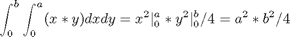Let's evaluate the integral numerically now with dblquad and compare the answer to the analytic one. I am using the default tolerance and the quadl method for integration.

tol = [];
qexact = (a^2 * b^2) / 4
qnumeric =
36
qexact =
36


### Elliptical Domain

The equation for an ellipse can be written as: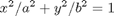and we can rewrite y as a function of x for the upper-right quadrant as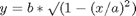We can now write the equation for the ellipse's area by integrating the identity integrand from 0 to the right limit for each value of x.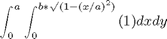and now we are ready to set up a function handle representing the inner integral. Let's plot the function.

fh = @(x) quadl(@(y) ones(size(y)), 0, b*sqrt(1-(x/a).^2));

x = 0:a/100:a;
ell = arrayfun(fh, x);
plot(x,ell); title('Ellipse boundary in first quadrant'); axis equal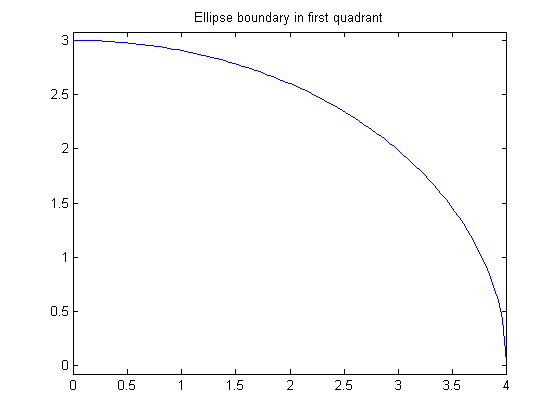The reason I used arrayfun to evaluate fh above is the same reason why I can't just use quadl for the outer integral. fh is not a vectorized function and I can't make it into one. To evaluate fh for each value of x, I am calculating an integral at each point and the limit of integration is a function of x. In addition, for each value of x, the integration method stops when the solution meets the correct numerical criteria and therefore each integration may not be performing the same number of function evaluations, nor will they necessarily evaluate the separate integrands at the same locations.

Simply calling quadl with the function defined by fh above won't work for the same reasons that I couldn't just vectorize my function evaluations of the integrand. Instead, we need an integration method that doesn't assume the integrand is vectorized. And that's what quadvec, the code I'm going to show you next, does (and it's similar to the inner workings of dblquad as well).

dbtype quadvec
1     function q = quadvec(f, varargin)
3     %   QUADVEC has the same calling syntax as QUAD but doesn't require that
4     %   the integrand be vectorized.  This makes QUADVEC suitable to perform
5     %   double-, triple-, and higher order integrals over a non-rectangular
6     %   domain.
7     %   Q = QUADVEC(FUN,A,B) tries to approximate the integral of scalar-valued
8     %   function FUN from A to B to within an error of 1.e-6 using recursive
10    %
11    %   Example:
12    %   Compute area of ellipse by computing the result in one quadrant and
13    %   then multiplying by 4.  Note that the inner integrand has integration
14    %   limits that are a function of the semi-major and semi-minor ellipse
15    %   axes, a and b.
16    %   First we set up the function handle to the inner integrand, fh.
17    %   a = 4; b = 3;
18    %   fh = @(x) quadl(@(y) ones(size(y)), 0, b*sqrt(1-(x/a).^2));
19    %   ea = 4 * quadvec(fh , 0, a);
20    %   ea-pi*a*b  % compare integration result to closed-form solution
21
22      q = quadl(@g, varargin{:}); % like quadl, but supplies g as the argument
23      function y = g(X) % make f into a "vectorized" function
24        y = zeros(size(X));
25        for i = 1:numel(X)
26          y(i) = f(X(i)); % this f refers to the argument of quadvec
27        end
28      end
29    end



You can see that we make sure we call the integrand for each value separately and we achieve this by making our function handle "vectorized" by creating a function handle to a nested function, and using this new function, g as the integrand. Looking at g, you can see, starting on line 25, the we use a for loop to iterate over all the input values.

We're now ready to calculate the ellipse's area. Remember, since we are only working in one quadrant, we need to multiply the result by 4.

ellipseArea = 4 * quadvec(fh,0,a)
ellipseArea =
37.6991


and we compare this to the analytic formula: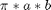ellipseAreaAnalytic = pi*a*b
ellipseAreaAnalytic =
37.6991


Do a little final housekeeping.

warning(s);

### Summary

Today I showed you how to perform integration in higher dimensions in MATLAB with the introduction of quadvec. You can see that quadvec ensures that we call the integrand one element at a time to allow us to cover cases in which the integrand isn't vectorized. Note that you can use quadvec on an integrand in 1 dimension, but performance will probably be worse than using quadl, assuming the integrand is vectorized.

Published with MATLAB® 7.2